## Wednesday, October 30, 2013

### Matrix Calculations: det(A - B * λ)=0

Let A and B be square matrices. B is a diagonal matrix. A diagonal matrix is a matrix with entries in it's diagonal positions, (1,1), (2,2), and so on, and 0 everywhere else. The identity matrix is a diagonal matrix. Another example is:

[ [2, 0, 0],[0, -2, 0],[0, 0, 5] ]

Let m, n, r, s, and t be numerical constants. Let a1, a2, and a3 be entries on the first row. Similar for b1, b2, b3, c1, c2, and c3.

det(A - B * ident(λ)) = 0

Comparison of 2 x 2 Matrices:
Note the constant term (λ^0), typed in green, the same.

B is a 2 x 2 identity matrix.

det( [ [a1, a2],[b1, b2] ] - λ * [ [1,0],[0,1] ] ) = 0
det( [ [a1, a2],[b1, b2] ] - [ [λ, 0],[0, λ] ]) = 0
λ^2 - (a1 + b2) * λ + a1*b2 - a2*b1 = 0

B is a diagonal matrix [ [m, 0],[0, n] ].

det( [ [a1, a2],[b1, b2] ] - λ * [ [m,0],[0,n] ] ) = 0
det( [ [a1, a2],[b1, b2] ] - [ [m*λ, 0],[0, n*λ] ]) = 0
m*n*λ^2 - (a1*n + b2*m)*λ + (a1*b2 - a2*b1) = 0

Comparison of 3 x 3 Matrices:
Note the constant term (λ^0), typed in green, the same.

B is a 3 x 3 identity matrix.

det( [ [a1,a2,a3],[b1,b2,b3],[c1,c2,c3] ] - λ * [ [1, 0, 0],[0, 1, 0],[0, 0, 1] ] ) = 0
det( [ [a1,a2,a3],[b1,b2,b3],[c1,c2,c3] ] - [ [λ, 0, 0],[0, λ, 0],[0, 0, λ] ] ) = 0

- λ^3
+ λ^2 * (a1 + b2 + c3)
+ λ * (-a1*b2 - a1*c3 - b2*c3 + a2*b1 + a3*c1 + b3*c2)
+ (a1*b2*c3 - a1*b3*c2 - a3*b2*c1 - a2*b1*c3 + a2*b3*c1 + a3*b1*c2) = 0

B is a general 3 x 3 diagonal matrix, [ [r, 0, 0],[0, s, 0],[0, 0, t] ].

det( [ [a1,a2,a3],[b1,b2,b3],[c1,c2,c3] ] - λ * [ [r, 0, 0],[0, s, 0],[0, 0, t] ] ) = 0
det( [ [a1,a2,a3],[b1,b2,b3],[c1,c2,c3] ] - [ [r*λ, 0, 0],[0, s*λ, 0],[0, 0, t*λ] ] ) = 0

- λ^3 * r*s*t
+ λ^2 * (s*t*a1 + r*t*b2 + r*s*c 3)
+ λ * (a1*b2*c3 - a1*b3*c2 - a3*b2*c1 - a2*b1*c3 + a2*b3*c1 + a3*b1*c2) = 0

Of course to get the eigenvalues (λ), solve the appropriate polynomial.

Hope this is helpful to those studying linear algebra or for those who are curious. This post was inspired from a program I was helping a fellow HP Prime programmer.

This post is dedicated to Michael de Estrada.

This blog is property of Edward Shore. 2013

## Saturday, October 26, 2013

### HP Prime Programming Tutorial #2: MSGBOX, IF-THEN-ELSE, PRINT, FOR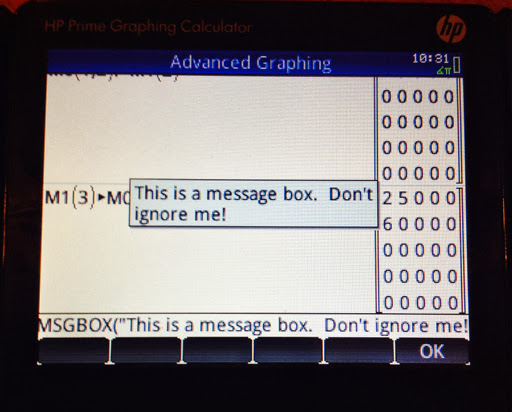Welcome to another programming tutorial for the HP Prime. In this session, we will cover MSGBOX, IF-THEN-ELSE, PRINT, and the FOR loop.

MSGBOX

MSGBOX: MSGOX takes a string a makes a pop-up message box. Program execution stops until you press a key to acknowledge the message.
Access: Cmds, 6. I/O, 8. MSGBOX

The program COMLOCK: Imagine that you are in charge of setting the combinations for the good, old-school combination locks. This program gives three digit combinations through the use of MSGBOX.

EXPORT COMLOCK()
BEGIN
LOCAL L0;
L0:=RANDINT(3,0,39);**
MSGBOX("SECRET: "+L0(1)+","+L0(2)+","+L0(3));
END;

** Thanks to Thomas Lake for pointing out my typo.  Apologies for any inconvenience - Eddie (3/21/2014)

Other commands that are featured:

RANDINT(n, a, b) generates a list of n integers between a and b. You can leave n out if you desire a single random integer. Picks may be repeated.

The HP Prime's default list variables are designated L0 through L9.

Here is a sample output for COMLOCK:IF-THEN-ELSE

IF-THEN-ELSE: Program structure:
IF condition THEN
do if the condition is true;
ELSE
do if the condition is false;
END;

Access: Tmplt, 2. Branch, 2. IF THEN ELSE

Tip: You can leave out the ELSE part if you only want to test to see if a condition is true. Access the simple IF-THEN structure by pressing Tmplt, 2. Branch, 1. IF THEN.

Access <, ≤, ==, etc. by pressing Shift, 6. Note that the double equals is needed to check equality.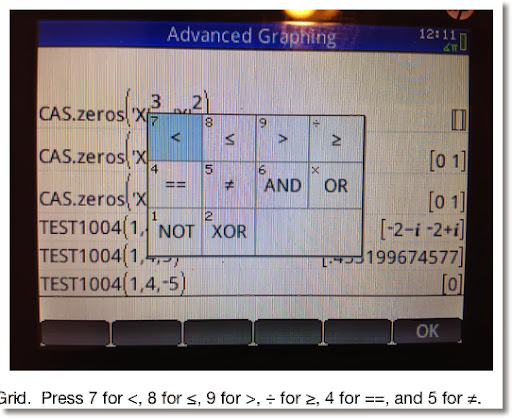PRINT

PRINT: The PRINT command prints a sting, result, or a combination of both onto the Prime's Terminal screen. If PRINT is used, the program will end on the terminal (text output) screen. Press a button to exit.

You can access the terminal screen at any time by pressing the ON button, holding it, and then pressing the Divide ( ÷ ) button.

Access: Cmds, 6. I/O, 9. PRINT

Tip: To clear the terminal screen, type PRINT(). This is a good way to clear the terminal screen and I usually use this at the beginning of any program if PRINT is going to be used later on.

The program QROOTS (yet one more quadratic solver, sorry for not being original guys and gals), demonstrates the use of IF-THEN-ELSE and PRINT.

Here I set the setting variable HComplex to 1, which allows for complex number results.

EXPORT QROOTS(A,B,C)
BEGIN
LOCAL D;
PRINT();
HComplex:=1;
D:=B^2-4*A*C;
IF D≥0 THEN
PRINT("Roots are real.");
ELSE
PRINT("Roots are complex.");
END;
PRINT((-B+√D)/(2*A));
PRINT((-B-√D)/(2*A));
END;

Examples:

QROOTS(1,5,8) returns:

Roots are complex.
-2.5+1.32287565553*i
-2.5-1.32287565553*i

QROOTS(2,-4,-8) returns:

Roots are real.
3.2360679775
-1.2360679775

FOR

This section will explore the basic FOR structure:

FOR variable FROM start TO end DO
commands;
END;

All the commands in the loop will be executed a set number of times. Each time a loop finishes, the variable increases by one. The loop terminates when variable=end.

Access: Tmplt, 3. LOOP, 1. FOR

The program SUMDIV takes any integer and adds up the sum of its divisors. For example, the divisors of 12 are 1, 12, 2, 3, 4, and 6. The sum is 28.

Featured Commands in SUMDIV:

idivis: idivis(integer) returns a sequence of all of the divisors if integer. Access: Toolbox, CAS, 5. Integer, 1. Divisors

Any CAS command used in programming will be preceded by "CAS." Not all CAS commands can be used in HP Prime programming at this time.

DIM: returns the dimensions of a sequence, string, or matrix. DIM must be used instead of SIZE to prevent a Bad Argument error.

For sequences or vectors, DIM returns the length in a list {length}.
For strings, DIM returns length as a number.
For matrices, DIM returns the list {number of rows, number of columns}.

Access: Cmds, 1. Strings, 9. DIM

The program:

EXPORT SUMDIV(N)
BEGIN
LOCAL S:=0,K,mdiv,ldiv;
mdiv:=CAS.idivis(N);
ldiv:=DIM(mdiv);
FOR K FROM 1 TO ldiv(1) DO
S:=S+mdiv(K);
END;
RETURN S;
END;
** Thanks to Thomas Lake for pointing out that the variable "mat", which I had in this program was unnecessary.  - Eddie 3/21/2013

Examples:
SUMDIV(12) returns 28.
SUMDIV(24) returns 60.
SUMDIV(85) returns 108.

This concludes this part of the HP Prime Programming Tutorial.

HAPPY HALLOWEEN!

Eddie

This blog is property of Edward Shore. 2013

## Thursday, October 24, 2013

### HP Prime Programming Tutorial #1: LOCAL, RETURN

Over the next month, maybe month and a half, I plan to post programming tutorials for the HP (Hewlett Packard) Prime.

If you have programmed with the HP 38G, 39g, or 39gii, this language will be similar to those. The programming language for the Prime is named the HP Prime Programming Language (HPPP).

Throughout this tutorial, I am going to use the latest version of the software.

How to start writing a program:

1. Press Shift + 1 (Program).
2. Press New. It is the second touch key.
3. Enter the name of the program. Pressing the ALPHA key twice will turn on UPPERCASE ΑLPHA-LOCK. Pressing ALPHA, Shift, ALPHA will turn on lowercase alpha-lock. To exit any lock, press the ALPHA key one more time. When happy with the name, press Enter.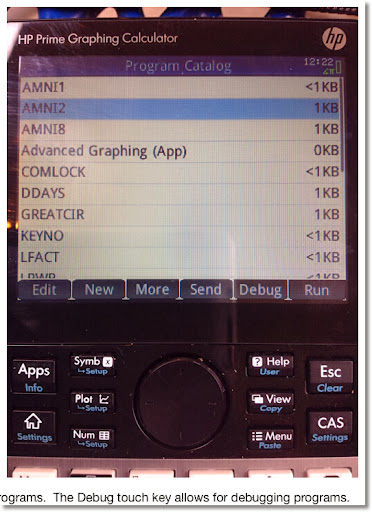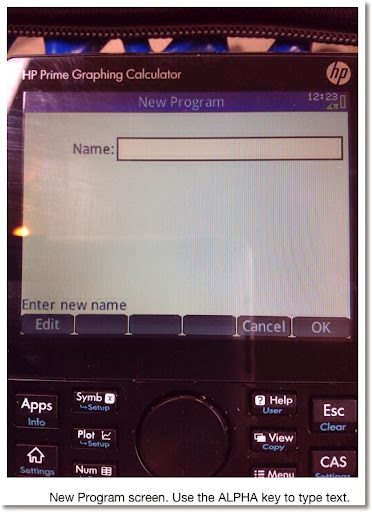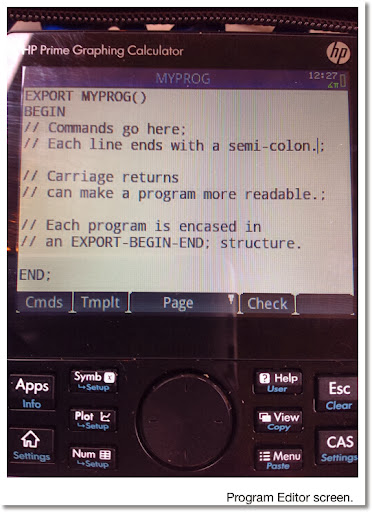Rules for Program Names:

1. Letters, numbers, and the underscore character (_) only.

Structure of a HP Prime Program

A HPPP program is encased of an EXPORT - BEGIN - END structure. The layout is generally like this:

EXPORT program_name(arguments)
BEGIN
END;

Each line containing a command generally must end with a semicolon (;). A semicolon can by type by pressing ALPHA then the Plus key ( + ).

Comments can be typed. The are designated by two forward slashes. The slashes are typed by pressing the Divide key ( ÷ ). Anything in the line following the two slashes is ignored in running the program.

SQIN

Our first program is SQIN, because "Hello World" programs are so 2000s. SQIN takes a number, squares it, then calculates the reciprocal. In short we are defining a custom function:

SQIN(x) = 1/x^2

Commands:

RETURN: returns a result to the stack (home page). You can return numbers, lists, vectors, matrices, strings, or a combination of these times.
Access: Tmplt, 1. Block, 2. RETURN

All the program code in this tutorial series will be shown in Courier font.

EXPORT SQIN(X)
BEGIN
RETURN 1/X^2;
END;

Tip: You can check the syntax of the program just by pressing the Check soft key in the program editor. HP Prime will inform you if there is a syntax error and attempt to point you to the error. If there are no syntax errors, the Prime states "No errors in the program". I use the Check command all the time.

How to run the programs:

Home Mode - Textbook Entry,
Home Mode - Algebraic Entry,
CAS Mode:

Type the program name. Follow the name with parenthesis and enclose the required arguments.

Or use the Toolbox (top row of white keys, 2nd key from the left, it looks like a tool box), select the User touch key, select the program, and input the required arguments.

Home Mode - RPN Entry:

Enter each argument, separate each entry by pressing the Enter key. Type the name, and in the parenthesis state the number of arguments.

For example, if the program TEST has four arguments, the RPN stack would like this:

4: argument_1
3: argument_2
2: argument_3
1: argument_4
TEST(4) to run the program.

Examples to try with SQIN:

SQIN(5) returns .04
SQIN(36) returns .000771604938

The next program will demonstrate the concept of local variables.

MOPMT

LOCAL: Declares any variables to be local to the program. In other words, the variables are created, used, possibly displayed during program execution, and deleted at program termination.
Access: Tmplt, 4. Variable, 1. LOCAL

Tip: You can declare local variables and assign an initial value at the same time. For example: LOCAL K:=1; stores 1 in K and makes K a local variable.

MOPMT calculates the monthly payment of a loan. The arguments are: the loan amount (L), the interest rate (R), and the number of months (M).

EXPORT MOPMT(L,R,M)
BEGIN
LOCAL K:=R/1200;
K:=L*K/(1-(1+K)^-M);
RETURN "Payment ="+K;
END;

Tip: Use RETURN, TEXTOUT_P, and PRINT to return custom strings, which combine results, messages, and calculations. Parts are connected with a plus sign.

Examples:
MOPMT(4000, 9.5, 30) returns 150.317437565
MOPMT(370000, 3.5, 360) returns 1661.46534383

Try this and next time in the series I will highlight other things we can do with HPPP. Thanks!

Eddie

This blog is property of Edward Shore. 2013

## Friday, October 18, 2013

### Program: Operations with Large Numbers (HP Prime)

Operations with Large Numbers

Large Factorial (Version 2) - HP Prime

EXPORT LFACT(N)
BEGIN
LOCAL K,S,A;
FOR K FROM 2 TO N DO
S:=S+LOG(K);
END;
RETURN { ALOG(FP(S)), IP(S) };
END;

This is an approximation! Results: {mantissa, exponent}. To be interpreted like this: mantissa * 10^exponent

Examples (compared with actual calculations with HP 50g):

55!
LFACT: 1.2696403353 * 10^73
Actual: 1.26964033537 * 10^73

65!
LFACT: 8.24765059247 * 10^90
Actual: 8.24765059208 * 10^90

75!
LFACT: 2.48091408072 * 10^109
Actual: 2.48091408114 * 10^109

125!
LFACT: 1.88267716466 * 10^209
Actual: 1.88267717689 * 10^209

245!
LFACT: 3.44638099725 * 10^480
Actual: 3.44638108855 * 10^480

525!
LFACT: 6.89080261728 * 10^1201
Actual: 6.89080262404 * 10^1201

875!
LFACT: 1.31677044437 * 10^2196
Actual: 1.31677038751 * 10^2196

Large Powers - HP Prime

EXPORT LPWR(X,Y)
BEGIN
LOCAL S;
S:=Y*LOG(X);
RETURN { ALOG(FP(S)), IP(S) };
END;

This is an approximation! Results: {mantissa, exponent}. To be interpreted like this: mantissa * 10^exponent

Examples (compared with actual calculations with HP 50g):
50^38
LPWR: 3.63797880819 * 10^64
Actual: 3.63797880709 * 10^64

102^68:
LPWR: 3.84425050422 * 10^136
Actual: 3.84425050254 * 10^136

58^124:
LPWR: 4.624568333 * 10^218
Actual: 4.62456834156 * 10^218

302^219:
LPWR: 1.32288017481 * 10^543
Actual: 1.32288017363 * 10^543

This blog is property of Edward Shore. 2013

## Tuesday, October 15, 2013

### Calculus Lesson: Area Between Curves

Area Between Two Curves

The general formula for finding the area between two curves is:

b
∫ T(x) - B(x) dx
a

I named the functions T(x) and B(x) specifically. T(x) represents the function "on top", while B(x) represents the function "on the bottom". In short, for x ∈ (a,b), T(x) ≥ B(x).

Here is an approach to use when finding areas between curves. A graphing calculator or mathematical software can be helpful in this procedure.

1. Draw the graphs. This is where a graphing calculator comes in handy.

2. Determine a and b, your limit points. Sometimes one or both of the limit points are found by finding the intersection of the two curves. Other problems will give you a third (or even fourth) condition. An example is x≥0, which makes one of our limit points x=0.

3. Determine which of the curves is T(x) and B(x). T(x) has greater numerical value than B(x) over the interval x ∈ (a, b).

4. Take the definite integral of T(x) - B(x). The result is your area.

b
∫ T(x) - B(x) dx = Area
a

About the examples and problem set: Please note that most examples in math textbooks, the numbers come out "nice and neat". Not so here, so I am using an HP Prime to demonstrate these examples and come up with answers. (any graphing calculator, HP, TI, Casio, all will do just fine!)

Example 1:

Find the area between:
y = -x^2 + 2
y = x^2 + 1

Here is a graph of the two curves: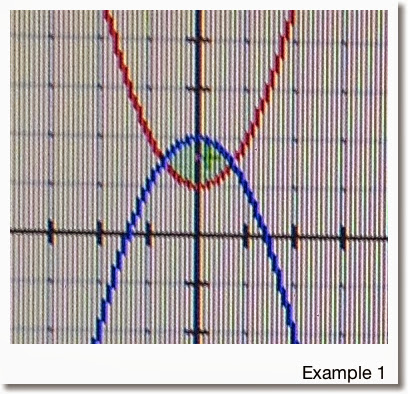Finding the limit points:

-x^2 + 2 = x^2 + 1
1 = 2*x^2
1/2 = x^2
Which implies x = -1/√2 and x = 1/√2

So our limit points are a = -1/√2 and b = 1/√2

From the graph we note that:
T(x) = -x^2 + 2
B(x) = x^2 + 1
and
T(x) - B(x) = -x^2 + 2 - (x^2 + 1) = -2*x^2 + 1

Taking the integral:

1/√2
∫ -2*x^2 + 1 dx ≈ 0.94281
-1/√2

So the area for Example 1 is about 0.94281.

Example 2:

Find the area between the two curves:
y = 2 cos x
y = x - 2
With the condition x≥0

First we graph the two curves: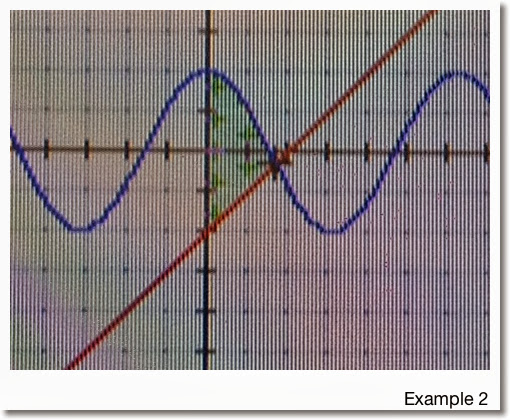The two graphs intersect when x>0. Since we are given the additional condition that x≥0, the lower limit for this problem is a=0.

To find the upper limit, we find intersection point:
2 cos x = x - 2
2 cos x - x + 2 = 0

Using a numeric solver on a calculator, we find that the intersection point is x ≈ 1.71419. (See, the numbers in these examples aren't always as "neat").

In the region x ∈ (0, 1.71419), we see that 2 cos x > x - 2. Hence:

T(x) = 2 cos x
B(x) = x - 2
and the area is approximately

1.71419
∫ 2 cos x - (x + 2) dx ≈ 3.93863
0

Problems to Try

Problem 1
Find the area between the curves of
y = x^2
y = 4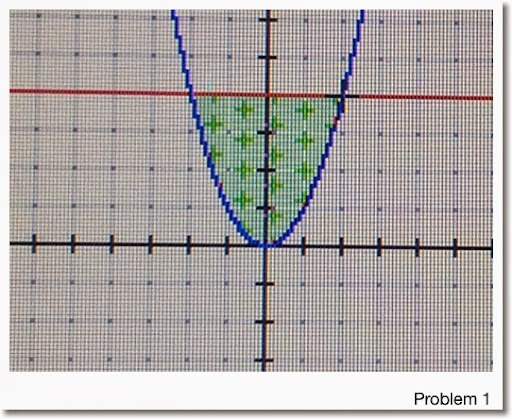Problem 2

y = x^3
y = -3*x + 8
With the condition x≥0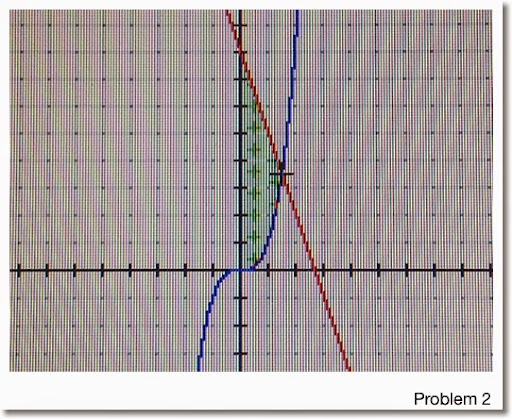Problem 3

y = 8*x^2 + 4*x - 6
y = x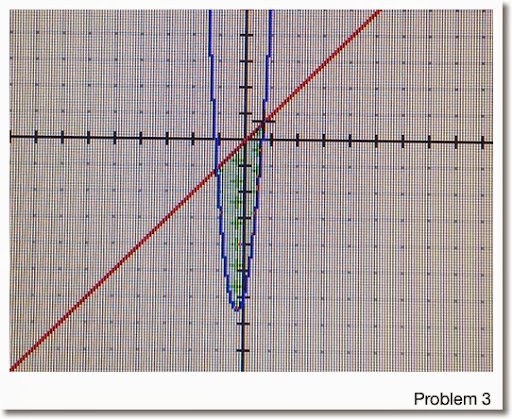Areas are rounded to five decimal points:
1. 10.66667
2. 7.36017 (Hint: Upper Limit ≈ 1.51275)
3. 7.42101 (Hint: Use a calculator to find the limit points)

Any questions, please leave them in the comment box. Hope this is helpful and thanks!

Eddie

This blog is property of Edward Shore. 2013

## Sunday, October 13, 2013

### HP 35S: Resistors in Series or Parallel (Portred from HP-33E)

Resistors in Series or Parallel
Source: HP-33E Student Engineering Applications, 1978
Original Calculator: HP-33S
Credit to Hewlett Packard Company
Ported to HP 35S by Eddie Shore, 10/10/2013

Instructions:
1. For each group of parallel or series resistors: press XEQ R.
2. At the Mode Prompt (INPUT M), enter 0 R/S for Parallel Resistors, 1 R/S for Series Resistors
3. Enter resistor values (assumed to be in Ohms) then press R/S.
4. To find the total, press XEQ R016.
5. Optional: Store the result in a variable for future use, as long is it not R.

Parallel Resistors: total resistance = 1/( 1/R1 + 1/R2 + ... )

Series Resistors: total resistance = R1 + R2 + ...

Program:
R001 LBL R
R002 0
R003 STO R
R004 SF 10 // press Left Shift, Flags, SF, decimal point, 0
R005 0=PAR 1=SER // equation
R006 PSE
R007 CF 10 // press Left Shift, Flags, CF, decimal point, 0
R008 INPUT M
R009 R/S
R010 RCL M
R011 x=0?
R012 XEQ R024
R013 R-down
R014 STO+ R
R015 GTO R009

R016 RCL R // total resistance
R017 RCL M
R018 x=0?
R019 XEQ R024
R020 CLx
R021 STO M
R022 R-down
R023 RTN

R024 R-down
R025 1/x
R026 ENTER
R027 RTN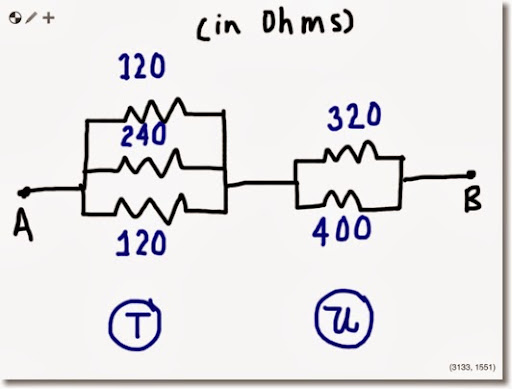Example 1:

Keystrokes:
1st Set:
XEQ R ENTER
0 R/S
120 R/S
240 R/S
120 R/S
XEQ R016
STO T

2nd Set:
XEQ R ENTER
0 R/S
320 R/S
400 R/S
XEQ R016
STO U
RCL+ T

Total Resistance: 225 7/9 ohms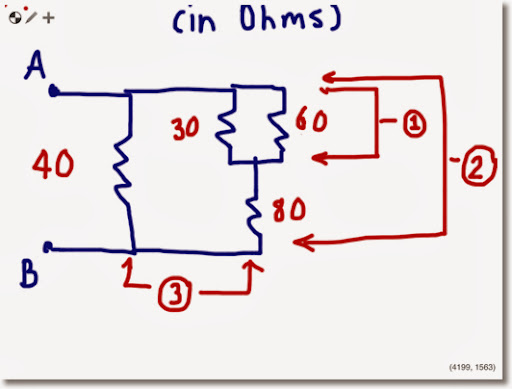Example 2:

XEQ R ENTER
0 R/S
30 R/S
60 R/S
XEQ R016 // Result: 20
STO T

XEQ R ENTER
1 R/S
RCL T R/S
80 R/S
XEQ R016 // Result: 100
STO T

XEQ R ENTER
0 R/S
RCL T R/S
40 R/S
XEQ R016

Total Resistance: 28 4/7 ohms

### Interesting Base Conversions - Porting a 1975 HP 25 Program to the HP 35S

Sometimes simple is best.

Base N to Base 10
Source: HP 25 Applications, 1975, pg. 22
Credit to Hewlett Packard Company
10/8/2013

Instructions:
Store the base of the number to be converted in B.
Start with the left most integer, press XEQ B. For each successive integer, press R/S. This is the integer portion.
Start with the left most fractional part, press XEQ B016. For each successive fractional digit, press R/S.

Examples:
1777 base 8 → 1023
143.2044 base 5 → 48.4384
1.11011 base 2 → 1.84375
B3A.F base 16 → 3131.9375

Program:
B001 LBL B
B002 STO A
B003 RCL B
B004 ENTER
B005 ENTER
B006 ENTER
B007 RCL A
B008 R/S
B009 STO A
B010 CLx
B011 +
B012 x
B013 RCL A
B014 +
B015 GTO B008
B016 RCL B
B017 1/x
B018 STO C
B019 STO D
B020 x
B021 R/S
B022 RCL C
B023 RCL D
B024 x
B025 STO D
B026 x
B027 +
B028 GTO B021

Base 10 to Base N
Source: HP 25 Applications, 1975, pg. 24
Credit to Hewlett Packard Company
10/8/2013

Instructions:
1. Store the number to be converted in A
2. Store the desired base in B
3. Press R/S stop. The approximation will build and keep building indefinitely until stopped.

Examples:
67.32 → 4 03. 05 01 14 11 base 16 (≈43.51EA base 16)
π ≈ 11.001001 base 2

√2 ≈ 1.0110101 base 2

Program:
T001 LBL T
T002 RCL B
T003 10
T004 x≥y?
T005 GTO T007
T006 100
T007 STO C
T008 0
T009 STO D
T010 RCL A
T011 LN
T012 RCL B
T013 LN
T014 ÷
T015 x<0?
T016 GTO T019
T017 IP
T018 GTO T022
T019 IP
T020 1
T021 -
T022 STO E
T023 RCL C
T024 x<>y
T025 y^x
T026 RCL D
T027+
T028 STO D
T029 PSE
T030 RCL B
T031 RCL E
T032 y^x
T033 STO - A
T034 GTO T010

This blog is property of Edward Shore. 2013

## Thursday, October 10, 2013

### Jeppesen E68 Wind Easy Computer

This past Sunday at the Pasadena City College Swap Meet, I found and bought a Jeppensen E6B Wind-Easy Computer. In reality, the E6B-2A is a slide rule specialized for aviation. The box may have not been in the best shape, how every he slide rule, its metal insert, instruction manual, and carrying pouch are all in great condition.

The E6B-2A has two sides: a calculator side and a wind side.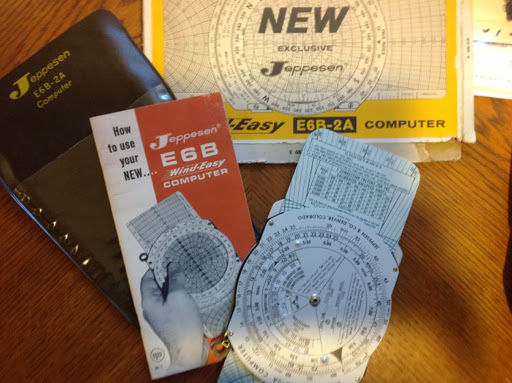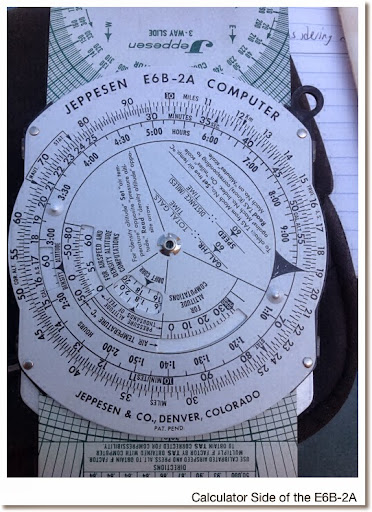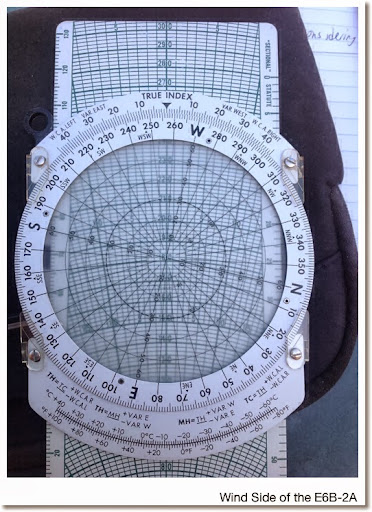Though my schedule has not allowed me much time to work with the E6B-2A, from the manual each of the two sides of the slide rule allows for specific calculations.

Calculator Side:
* Computation of speed, distance, and time
* Distance conversions: kilometers, miles, and nautical miles, which each of the measuring guides marked on the outside scale.
* Altitude Calculations: Density and True Altitude
* True Air Speed
* Mach Speed
* Slide rule, where the outer and inner scales act as scales C and D, respectively.

Wind Side:
* Calculations involving the "wind triangle", made up of the wind vector, ground vector, and air vector.
* Using the wind charts to plot true speed and course.

I have never owned a slide rule like this before, very interesting and unique - and something to carve out some time to learn. Very cool item!

Eddie

This blog is property of Edward Shore. 2013

.

## Wednesday, October 2, 2013

### HP Prime Program: Sampling Without Replacement

SAMPLE - Generate a list of random integers from 1 to N using sample without replacement.

Author: Eddie Shore
Date: 10/1/2013

Syntax: SAMPLE(L, N)
L = length of desired list
N = high integer
If L > N, an error occurs.

Output: random sample

Program:
EXPORT SAMPLE(L,N)
BEGIN
// length, number
LOCAL I, K, T, num;

// error cond
IF L > N THEN
1/0;
END;

// main program
L1:=MAKELIST(0,X,1,L,1);
L1(1):=RANDINT(N-1)+1;
I:= 2;

REPEAT
num:=RANDINT(N-1)+1;
T:=1;

// test for uniqueness
FOR K FROM 1 TO I DO
IF num == L1(K) THEN
T:=T + 1;
END;
END;

IF T == 1 THEN
L1(I) := num;
I := I + 1;
END;

UNTIL I == L + 1;
RETURN L1;
END;

Examples:
SAMPLE(5,9) (length of 5, n = 9) can generate:
{5, 4, 8, 2, 6}
{9, 7, 8, 1, 2}
{4, 3, 6, 5, 2}

This blog is property of Edward Shore. 2013

### HP Prime Program: ULAM

ULAM - Using Ulam's Conjecture to determine the number of steps it takes to reduce an integer N to 1 using the following rules:

If N is odd: N = 3×N+1
If N is even: N=N/2

9/24/2013

Program:

EXPORT ULAM(N)
BEGIN
LOCAL C;
PRINT();
REPEAT
IF FP(N/2)==0 THEN
N:=N/2;
ELSE
N:=3*N+1;
END;
C:=C+1;
PRINT(N);
UNTIL N==1;
RETURN C;
END;

Examples:
ULAM(69): 208, 104, 52, 26, 13, 40, 20, 10, 5, 16, 8, 4, 2, 1 (14 steps)

ULAM(84): 42, 21, 64, 32, 16, 8, 4, 2, 1 (9 steps)

This blog is property of Edward Shore. 2013

## Tuesday, October 1, 2013

### HP Prime Tip: Changing the Color of a Function

In the graphing apps of the HP Prime, like the Function, Advanced Graphing, Parametric, and Polar apps, expressions can be graphed in various colors.

There are two ways to change the colors of a graph. First, the easy, but limited way.

For this example, I am going to use the Function App with F1(X) = 2 * e^(SIN(X)). Assume the View is set to Autoscale.

1. Press the Symb key. To the left of the expression box is the color box. Move left until the color box is highlighted. You will know that the color box is highlighted when the screen says "Choose graph color".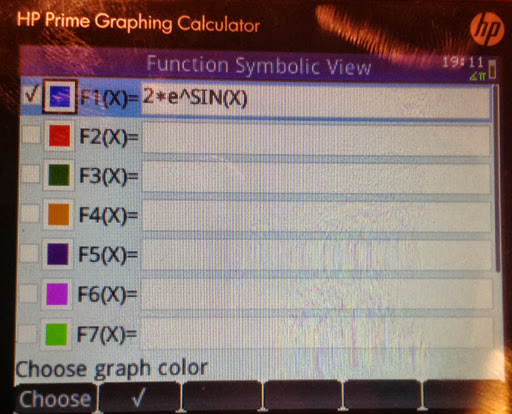2. Hit the Choose soft key. An array of nine colors will appear. Choose the desired color and press Enter. For this example I will select red.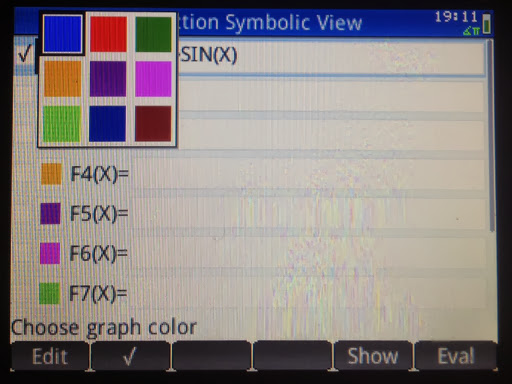Here is F1 graphed in red: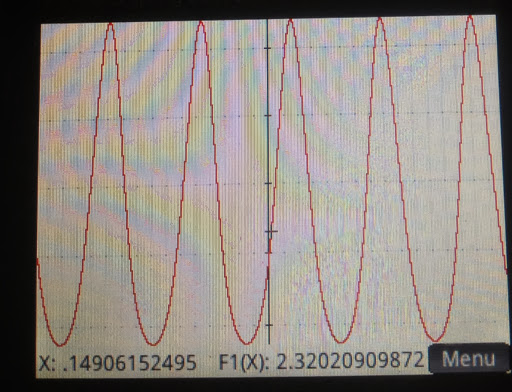However, what if you want to:
1. Graph the function in a color other than the nine presented in the Symbolic page? (This includes black)
2. Be able to change the color of the function in a program?

We can do that too, with the following:

F1(COLOR):=#RRGGBBh where RRGGBBH is a hexadecimal code, or
F1(COLOR):=RGB(red, green, blue)

You can type the command in either Home mode set to Textbook or Algebraic Entry or Program Editor mode.

Caution: Doing this in CAS mode will result in the color code stored in the function - not desirable.

If you are in Home Mode, RPN Entry, either:

2. Press Enter
3. Type 'F#(COLOR)' in single quotes. Press Enter. (use the appropriate name and type).
4. Press Shift+EEX (STO>).

or

1. Enter the red intensity, press Enter
2. Enter the green intensity, press Enter
3. Enter the blue intensity, press Enter
4. Execute RGB(3). In RPN mode, the 3 tells the Prime to take the last three stacks as the arguments of RGB.
5. Type 'F#(COLOR)' in single quotes. Press Enter. (use the appropriate name and type)
6. Press Shift+EEX (STO>).

The maximum values for each of the colors is 255, or FF in base 16. This is similar to the range of colors you can choose from on a typical personal computer program. 0 is the least intense, 255 is the most intense.

For a list of colors and their color codes, one of the web pages you can visit is http://www.rapidtables.com/web/color/Web_Color.htm. Scroll down to see a table that has both hexadecimal and RGB codes.

Let's demonstrate with F1(X) = 2 * e^(SIN(X)).

First graph F1 in black. The RGB code for black is RGB(0,0,0) or #00000h. I have the calculator set to Home Mode, Textbook Entry.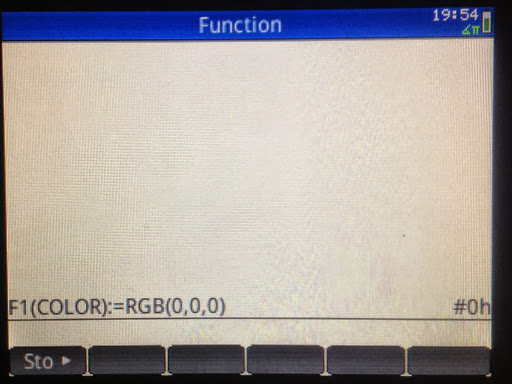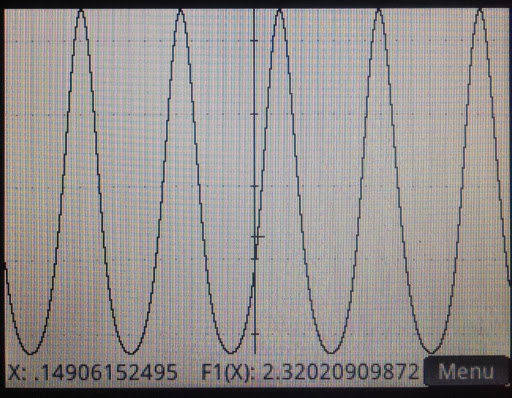Now plotting F1 in Dodger Blue, RGB(30,144,255) or #1E90FFh in Home Mode, RPN entry: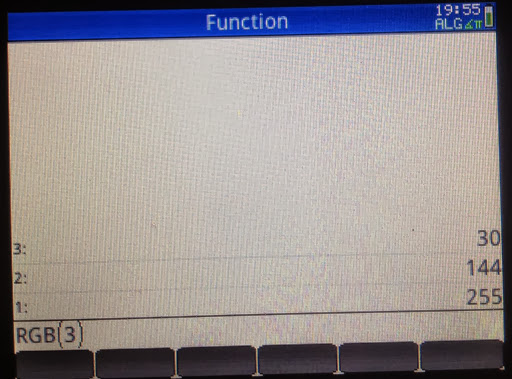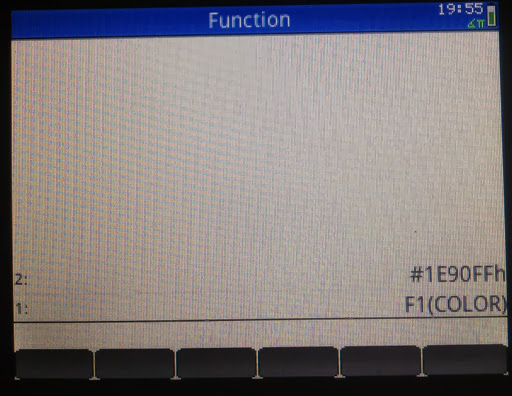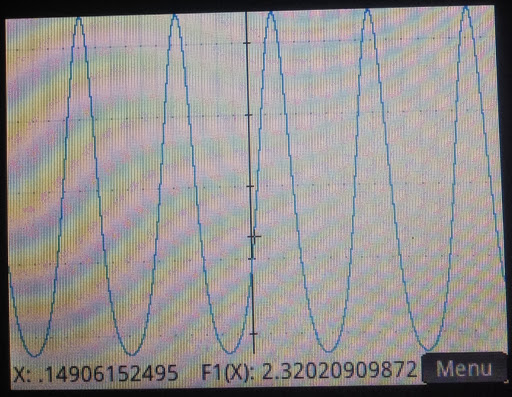That is how to plot in color. Until next time,

Eddie

This blog is property of Edward Shore. 2013

### HP 15C: Error Function and Lower Tail Normal Cumulative Function

HP 15C:   Error Function and Lower Tail Normal Cumulative Function Formulas Used  Error Function  erf(x) = 2 ÷ √π * ∫( e^(-t^2) dt, t = 0 t...